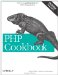# Recipe 4.4. Iterating Through an Array

#### 4.4.1. Problem

You want to cycle though an array and operate on all or some of the elements inside.

#### 4.4.2. Solution

Use foreach:

`foreach (\$array as \$value) {     // Act on \$value }`

Or to get an array's keys and values:

`foreach (\$array as \$key => \$value) {     // Act II }`

Another technique is to use for:

`for (\$key = 0, \$size = count(\$array); \$key < \$size; \$key++) {    // Act III }`

Finally, you can use each( ) in combination with list( ) and while:

`reset(\$array) // reset internal pointer to beginning of array while (list(\$key, \$value) = each (\$array)) {     // Final Act }`

#### 4.4.3. Discussion

A foreach loop is the most concise to iterate through an array:

`// foreach with values foreach (\$items as \$cost) {     ... } // foreach with keys and values foreach(\$items as \$item => \$cost) {     ... }`

With foreach, PHP iterates over a copy of the array instead of the actual array. In contrast, when using each( ) and for, PHP iterates over the original array. So if you modify the array inside the loop, you may (or may not) get the behavior you expect.

If you want to modify the array, reference it directly:

`reset(\$items); while (list(\$item, \$cost) = each(\$items)) {     if (! in_stock(\$item)) {         unset(\$items[\$item]);           // address the array directly     } }`

The variables returned by each( ) aren't aliases for the original values in the array: they're copies, so if you modify them, it's not reflected in the array. That's why you need to modify \$items[\$item] instead of \$item.

When using each( ), PHP keeps track of where you are inside the loop. After completing a first pass through, to begin again at the start, call reset( ) to move the pointer back to the front of the array. Otherwise, each( ) returns false.

The for loop works only for arrays with consecutive integer keys. Unless you're modifying the size of your array, it's inefficient to recompute the count( ) of \$items each time through the loop, so we always use a \$size variable to hold the array's size:

`for (\$item = 0, \$size = count(\$items); \$item < \$size; \$item++) {     ... }`

If you prefer to count efficiently with one variable, count backward:

`for (\$item = count(\$items) - 1; \$item >= 0; \$item--) {     ... }`

The associative array version of the for loop is:

`for (reset(\$array); \$key = key(\$array); next(\$array) ) {     ... }`

This fails if any element holds a string that evaluates to false, so a perfectly normal value such as 0 causes the loop to end early.

Finally, use array_map( ) to hand off each element to a function for processing:

`// lowercase all words \$lc = array_map('strtolower', \$words);`

The first argument to array_map( ) is a function to modify an individual element, and the second is the array to be iterated through.

Generally, we find this function less flexible than the previous methods, but it is well-suited for the processing and merging of multiple arrays.

If you're unsure if the data you'll be processing is a scalar or an array, you need to protect against calling foreach with a non-array. One method is to use is_array( ):

`if (is_array(\$items)) {     // foreach loop code for array } else {     // code for scalar }`

Another method is to coerce all variables into array form using settype( ):

`settype(\$items, 'array'); // loop code for arrays`

This turns a scalar value into a one-element array and cleans up your code at the expense of a little overhead.

Documentation on for at http://www.php.net/for, foreach at http://www.php.net/foreach, while at http://www.php.net/while, each( ) at http://www.php.net/each, reset( ) at http://www.php.net/reset, and array_map( ) at http://www.php.net/array-map .PHP Cookbook: Solutions and Examples for PHP Programmers
ISBN: 0596101015
EAN: 2147483647
Year: 2006
Pages: 445

Similar book on Amazon